Courses

SRMJEEE Chemistry Mock Test - 8

35 Questions MCQ Test SRMJEEE Subject Wise & Full Length Mock Tests | SRMJEEE Chemistry Mock Test - 8

Description
Attempt SRMJEEE Chemistry Mock Test - 8 | 35 questions in 50 minutes | Mock test for JEE preparation | Free important questions MCQ to study SRMJEEE Subject Wise & Full Length Mock Tests for JEE Exam | Download free PDF with solutions
QUESTION: 1

Solution:
QUESTION: 2

Solution:
QUESTION: 3

Compound (A)C₅H₁₀O forms a phenylhydrazone and gives negative Tollen's and iodoform test. Compound (A) on reduction gives n-pentane. Compound (A) is

Solution:
QUESTION: 4

When chlorine water is added to an aqueous solution of potassium halide in presence of chloroform, a violet colour is obtained. On adding more of chlorine water, the violet colour disappears, and a colourless solution is obtained. This test confirms the presence of the following in aqueous solution.

Solution:
QUESTION: 5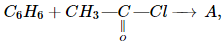Product A is

Solution:
QUESTION: 6

Chloride ion and potassium ion are isoelectronic. Then

Solution:
QUESTION: 7

Which of the following has a derived unit?

Solution:
QUESTION: 8

Carboxylic acids are more acidic than phenol and alcohol because of

Solution:
QUESTION: 9

Oleic, stearic, palmitic acids are

Solution:
QUESTION: 10

The hybrid orbitals at carbon 2 and 3 in the compound CH3CH = CHCH3 are :

Solution: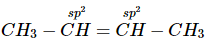QUESTION: 11

BCl3 molecule is planar while NCl3 is pyramidal because

Solution: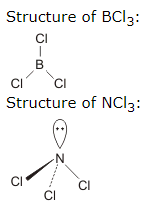QUESTION: 12

If a mixture containing 3 moles of hydrogen and 1 mole of nitrogen is converted completely into ammonia, the ratio of volumes of reactants and products at the same temperature and pressure would be

Solution:

3H2 + N2 → 2NH3
∴ 3V 1V → 2V
Total initial volume = 3V + 1V = 4V
Final volume = 2V
Hence, ratio of initial and final volume will be 2 : 1

QUESTION: 13

The law formulated by Nernst is

Solution:
QUESTION: 14

The time taken for 90% of a first order reaction to complete is approximately

Solution:
QUESTION: 15

Which of the following statements is true?

Solution:
QUESTION: 16

Medicine which is an antibiotic is

Solution:
QUESTION: 17

The number of πbonds in
CH₂ = CH - CH = CH - C ≡ CH is

Solution:
QUESTION: 18

Which of the following is isoelectronic as well as has the same structure as that of N₂O?

Solution:

CO2 and N2O are isoelectronic with 22 electrons and isostructural with linear geometry.
Structure of nitrous oxide: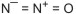Structure of carbon di-oxide:

O = C = O

QUESTION: 19

Which of the following will act as buffer solution ?

Solution:
QUESTION: 20

Baeyer's reagent is used in the laboratory for

Solution:
QUESTION: 21

The rms velocity at NTP of the species can be calculated from the expression

Solution:
QUESTION: 22

Which pair of isomerism is not possible together?

Solution:
QUESTION: 23

Which of the following solution cannot act as a buffer ?

Solution:
QUESTION: 24

In radioactive decay which one of the following moves the fastest ?

Solution:
QUESTION: 25

Which of the following is a strongest base?

Solution:
QUESTION: 26

An organic compound A reacts with methyl magnesium iodide to form an addition product which on hydrolysis forms the compound B. Compound B gives blue colour salt in Victor Meyer's test. The compounds A and B are respectively.

Solution:
QUESTION: 27

Which is most stable carbocation?

Solution:
QUESTION: 28

Polyvinyl alcohol can be prepared by

Solution:
QUESTION: 29

Lassaigne's test for the detection of nitrogen fails in

Solution:
QUESTION: 30

When iron is added to CuSO₄ solution, copper is precipitated. It is due to

Solution:
QUESTION: 31

A solid AB has NaCl type structure. If radius of the cation A is 100 pm, then radius of the anion is

Solution:
QUESTION: 32

Molal depression constant for water is 1.86°C. The freezing point of a 0.05 molal solution of a non electrolyte in water is

Solution:
QUESTION: 33

32 g of oxygen and 3.0 g of hydrogen are mixed and kept in a vessel at 760 mm pressure and 0°C. The total volume occupied by the mixture will be nearly

Solution:

Total volume of a mixture of gases is equal to the sum of the partial volume of the constituent gases.
V1 + V2 = Vtotal
while V1, that is, partial volume of a gas in a mixture is defined as the volume which the gas would occupy if it were present alone in a container at temperature T and pressure p of the mixture.
So, number of moles of O2 = 32/32 = 1 mole
number of moles of H2 = 3/2 = 1.5 moles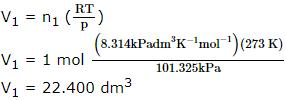similarly,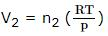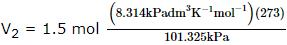V2 = 33.600 dm3
Hence the total volume occupied by the mixture = 56.000 dm3

QUESTION: 34

From a salt solution of Cu, 0.534 g of Mg displaces 1.415 g of Cu. If equivalent weight of Mg is 12, then equivalent weight of Cu will be

Solution:
QUESTION: 35

Collodial solution of arsenious sulphide is prepared by

Solution:Use Code STAYHOME200 and get INR 200 additional OFF Use Coupon Code

Track your progress, build streaks, highlight & save important lessons and more!

Similar ContentRelated tests